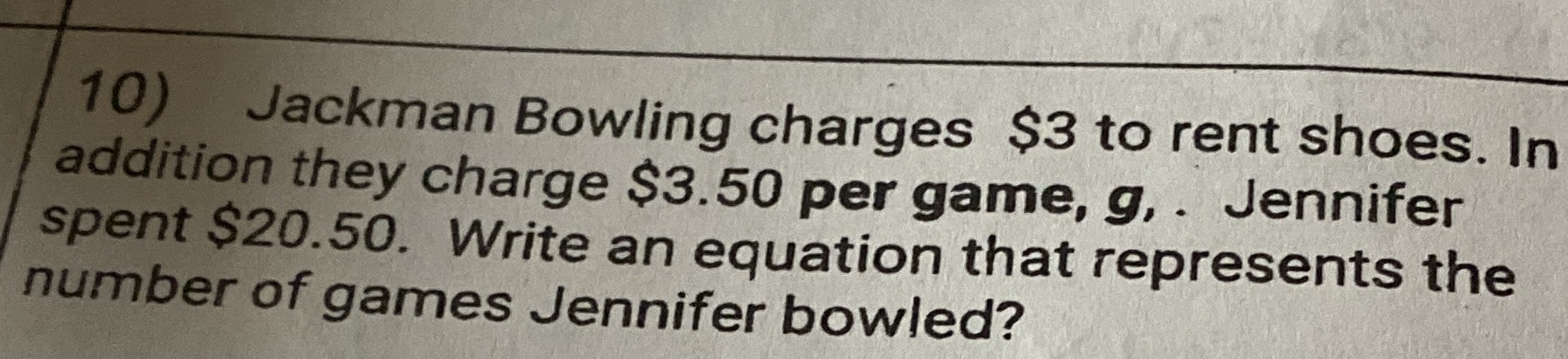### ¿Todavía tienes preguntas de matemáticas?

Pregunte a nuestros tutores expertos
Algebra
Pregunta10) Jackman Bowling charges $$\ 3$$ to rent shoes. In addition they charge $$\ 3.50$$ per game, $$g$$ . Jennifer spent $$\ 20.50$$ . Write an equation that represents the number of games Jennifer bowled?

$$3+ 3.5x= 20.5$$